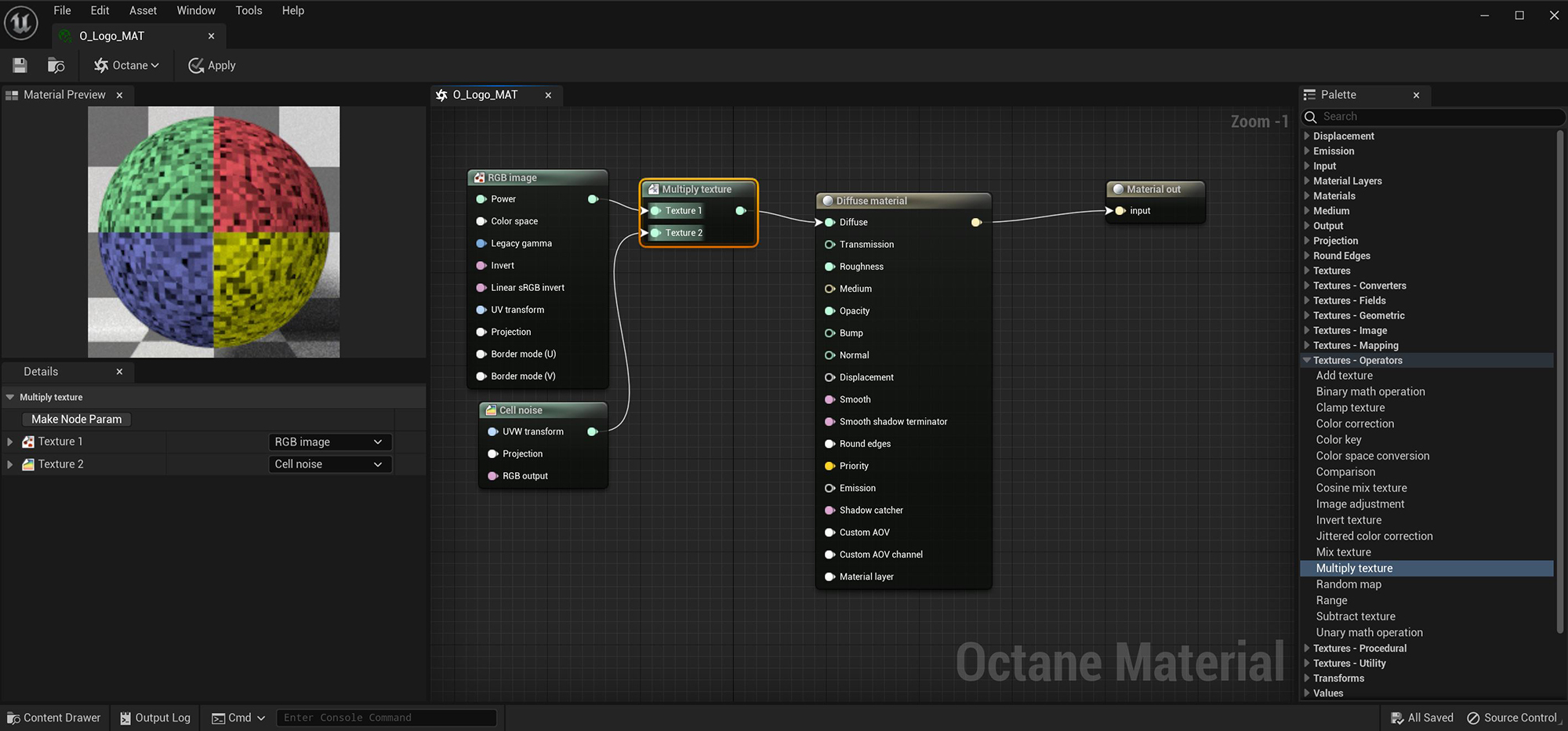### Multiply Texture

The Multiply texture multiplies Texture or Color values together in an overlay fashion (figure 1). The calculation is similar to the Multiply Layer mode used in Photoshop® that multiplies the color values of two layers.Figure 1: The Multiply texture node blends a RGB Image and Cell Noise texture together